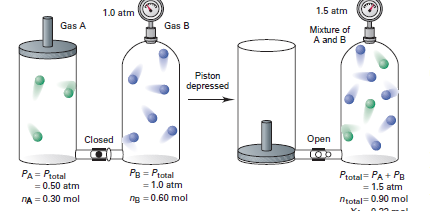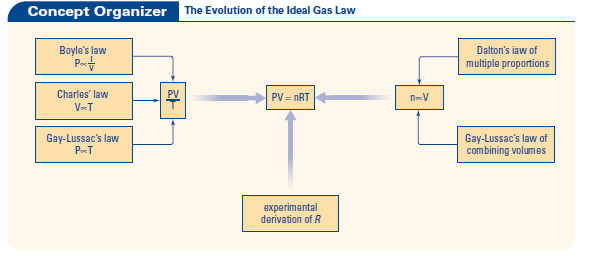# Pre University Courses

The image below shows an example of Dalton's Law of Partial Pressure. The total pressure of a mixture of gases is the sum of the pressures of each of the individual gases.What is the composition of the Earth's atmosphere?

 Components Percentage Nitrogen (N2 ) 78.08% Oxygen (O2 ) 20.95% Argon (Ar) 0.93 % Carbon Dioxide (CO 2 ) 0.03% Neon (Ne) 0.002 % Other Gases 0.008%

Question: If the atmosphere is 79% nitrogen, and the atmospheric Pressure is 101.3 kPa, what is the percentage partial contribution of nitrogen on this day?

Formula:  % of gas x pressure of the day

Complete: p. 22, 23, 24 in groups.

12.1 The Ideal Gas Law

Avogadro's hypothesis:  Equal volumes of all ideal gases at the same temperature and pressure contain the same number of molecules.

Avogadro's law gives us a mathematical relationship between the volume of a gas (V) and the number of moles of gas present (n)

n proportional to V

n=kV

n1= n2

V1    V2

Practice Problem p. 477, #1 and 2We combine all of the gas laws into the Ideal Gas law which is PV=nRT

R is the universal gas constant (R) and is 8.314 kPa L/mol K

This value is given at STP (Temperature is 0 degrees Celsius or 273 K and Pressure is 760 mmHg= 760 torr= 1 atm= 101.3 kPa) this is taken at sea level

The value of SATP               (Temperature is 25 degrees Celsius and Pressure 100 kPa) this is taken at normal standard ambient temperature and pressure.

p. 488, 12 and 13